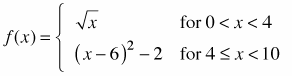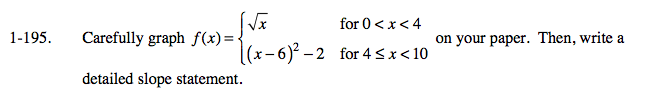### Home > CALC > Chapter 1 > Lesson 1.5.1 > Problem1-195

1-195.

Carefully graphon your paper. Then, write a detailed slope statement. 1-195 HW eTool (Desmos). Homework Help ✎Describe how the slope of the graph changes. Start at the beginning (x = 0) and end at the end (x = 10). Include where the slope is positive, where it is negative, where it is steep, where it is smooth, where it is increasing,where it is decreasing and where it is constant.

Use the eTool below to explore.
Click the link at right for the full version of the eTool: Calc 1-195 HW eTool